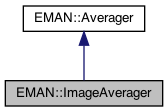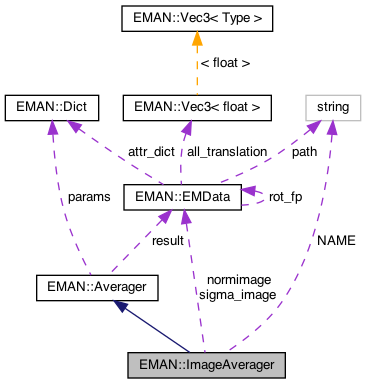EMAN2
EMAN::ImageAverager Class Reference

ImageAverager averages a list of images. More...

`#include <averager.h>`

Inheritance diagram for EMAN::ImageAverager:[legend]
Collaboration diagram for EMAN::ImageAverager:[legend]

## Public Member Functions

ImageAverager ()

To add an image to the Averager. More...

EMDatafinish ()
Finish up the averaging and return the result. More...

string get_name () const
Get the Averager's name. More...

string get_desc () const

TypeDict get_param_types () const
Get Averager parameter information in a dictionary. More...

virtual void mult (const float &)
Multiply the result image by some floating point constant This is useful when weighting the input images prior to calling add_image - a situation where it is likely you want to divide by the sum of the weights. More...Public Member Functions inherited from EMAN::Averager
Averager ()

virtual ~Averager ()

virtual void add_image_list (const vector< EMData * > &images)
To add multiple images to the Averager. More...

virtual void set_params (const Dict &new_params)
Set the Averager parameters using a key/value dictionary. More...

## Static Public Member Functions

static AveragerNEW ()

## Static Public Attributes

static const string NAME = "mean"

## Private Attributes

EMDatasigma_image

EMDatanormimage

int ignore0

int nimg

int freenormProtected Attributes inherited from EMAN::Averager
Dict params

EMDataresult

## Detailed Description

ImageAverager averages a list of images.

It optionally makes a sigma image.

Parameters
 sigma sigma value ignore0 if set, ignore zero value pixels

Definition at line 166 of file averager.h.

## ◆ ImageAverager()

 ImageAverager::ImageAverager ( )

Definition at line 221 of file averager.cpp.

222 : sigma_image(0), ignore0(0), normimage(0), freenorm(0), nimg(0)
223{
224
225}
EMData * sigma_image
Definition: averager.h:203
EMData * normimage
Definition: averager.h:203

Referenced by NEW().

## Member Function Documentation

 void ImageAverager::add_image ( EMData * image )
virtual

To add an image to the Averager.

This image will be averaged in this function.

Parameters
 image The image to be averaged.

Reimplemented from EMAN::Averager.

Definition at line 227 of file averager.cpp.

228{
229 if (!image) {
230 return;
231 }
232
233 if (nimg >= 1 && !EMUtil::is_same_size(image, result)) {
234 LOGERR("%sAverager can only process same-size Image",
235 get_name().c_str());
236 return;
237 }
238
239 nimg++;
240
241 int nx = image->get_xsize();
242 int ny = image->get_ysize();
243 int nz = image->get_zsize();
244 size_t image_size = (size_t)nx * ny * nz;
245
246 if (nimg == 1) {
248 result->set_size(nx, ny, nz);
249 sigma_image = params.set_default("sigma", (EMData*)0);
250 ignore0 = params["ignore0"];
251
252 normimage = params.set_default("normimage", (EMData*)0);
253 if (ignore0 && normimage==0) { normimage=new EMData(nx,ny,nz); freenorm=1; }
254 if (normimage) normimage->to_zero();
255 }
256
257 float *result_data = result->get_data();
258 float *sigma_image_data = 0;
259 if (sigma_image) {
260 sigma_image->set_size(nx, ny, nz);
261 sigma_image_data = sigma_image->get_data();
262 }
263
264 float * image_data = image->get_data();
265
266 if (!ignore0) {
267 for (size_t j = 0; j < image_size; ++j) {
268 float f = image_data[j];
269 result_data[j] += f;
270 if (sigma_image_data) {
271 sigma_image_data[j] += f * f;
272 }
273 }
274 }
275 else {
276 for (size_t j = 0; j < image_size; ++j) {
277 float f = image_data[j];
278 if (f) {
279 result_data[j] += f;
280 if (sigma_image_data) {
281 sigma_image_data[j] += f * f;
282 }
283 normimage->set_value_at_fast(j,normimage->get_value_at(j)+1.0);
284 }
285 }
286 }
287}
EMData * result
Definition: averager.h:158
type set_default(const string &key, type val)
Default setting behavior This can be achieved using a template - d.woolford Jan 2008 (before there wa...
Definition: emobject.h:569
EMData stores an image's data and defines core image processing routines.
Definition: emdata.h:82
static bool is_same_size(const EMData *image1, const EMData *image2)
Check whether two EMData images are of the same size.
Definition: emutil.cpp:1224
string get_name() const
Get the Averager's name.
Definition: averager.h:174
#define LOGERR
Definition: log.h:51

## ◆ finish()

 EMData * ImageAverager::finish ( )
virtual

Finish up the averaging and return the result.

Returns
The averaged image.

Implements EMAN::Averager.

Definition at line 289 of file averager.cpp.

290{
291 if (result && nimg > 1) {
292 size_t image_size = (size_t)result->get_xsize() * result->get_ysize() * result->get_zsize();
293 float * result_data = result->get_data();
294
295 if (!ignore0) {
296 for (size_t j = 0; j < image_size; ++j) {
297 result_data[j] /= nimg;
298 }
299
300 if (sigma_image) {
301 float * sigma_image_data = sigma_image->get_data();
302
303 for (size_t j = 0; j < image_size; ++j) {
304 float f1 = sigma_image_data[j] / nimg;
305 float f2 = result_data[j];
306 sigma_image_data[j] = sqrt(f1 - f2 * f2);
307 }
308
309 sigma_image->update();
310 }
311 }
312 else {
313 for (size_t j = 0; j < image_size; ++j) {
314 if (normimage->get_value_at(j)>0) result_data[j] /= normimage->get_value_at(j);
315 }
316 if (sigma_image) {
317 float * sigma_image_data = sigma_image->get_data();
318
319 for (size_t j = 0; j < image_size; ++j) {
320 float f1 = 0;
321 if (normimage->get_value_at(j)>0) f1=sigma_image_data[j] / normimage->get_value_at(j);
322 float f2 = result_data[j];
323 sigma_image_data[j] = sqrt(f1 - f2 * f2);
324 }
325
326 sigma_image->update();
327 }
328 }
329
330 result->update();
331
332 result->set_attr("ptcl_repr",nimg);
333
334 if (freenorm) { delete normimage; normimage=(EMData*)0; }
335 nimg=0;
336
337 return result;
338 }
339
340 return nullptr;
341}
EMData * sqrt() const
return square root of current image

References freenorm, ignore0, nimg, normimage, EMAN::Averager::result, sigma_image, and sqrt().

## ◆ get_desc()

 string EMAN::ImageAverager::get_desc ( ) const
inlinevirtual

Implements EMAN::Averager.

Definition at line 179 of file averager.h.

180 {
181 return "Simple mean average of images";
182 }

## ◆ get_name()

 string EMAN::ImageAverager::get_name ( ) const
inlinevirtual

Get the Averager's name.

Each Averager is identified by a unique name.

Returns
The Averager's name.

Implements EMAN::Averager.

Definition at line 174 of file averager.h.

175 {
176 return NAME;
177 }
static const string NAME
Definition: averager.h:200

References NAME.

## ◆ get_param_types()

 TypeDict EMAN::ImageAverager::get_param_types ( ) const
inlinevirtual

Get Averager parameter information in a dictionary.

Each parameter has one record in the dictionary. Each record contains its name, data-type, and description.

Returns
A dictionary containing the parameter info.

Reimplemented from EMAN::Averager.

Definition at line 189 of file averager.h.

190 {
191 TypeDict d;
192 d.put("sigma", EMObject::EMDATA, "sigma value");
193 d.put("normimage", EMObject::EMDATA, "In conjunction with ignore0, the number of non zero values for each pixel will be stored in this image.");
194 d.put("ignore0", EMObject::INT, "if set, ignore zero value pixels");
195 return d;
196 }

References EMAN::EMObject::EMDATA, EMAN::EMObject::INT, and EMAN::TypeDict::put().

## ◆ mult()

 virtual void EMAN::ImageAverager::mult ( const float & s )
inlinevirtual

Multiply the result image by some floating point constant This is useful when weighting the input images prior to calling add_image - a situation where it is likely you want to divide by the sum of the weights.

Hence call mult after all of the weighted images have been added.

Parameters
 s the scaling factor.
Exceptions
 NullPointerException if the EMData pointer (result) is NULL

Reimplemented from EMAN::Averager.

Definition at line 198 of file averager.h.

198{ }

## ◆ NEW()

 static Averager * EMAN::ImageAverager::NEW ( )
inlinestatic

Definition at line 184 of file averager.h.

185 {
186 return new ImageAverager();
187 }

References ImageAverager().

## ◆ freenorm

 int EMAN::ImageAverager::freenorm
private

Definition at line 206 of file averager.h.

## ◆ ignore0

 int EMAN::ImageAverager::ignore0
private

Definition at line 204 of file averager.h.

## ◆ NAME

 const string ImageAverager::NAME = "mean"
static

Definition at line 200 of file averager.h.

Referenced by get_name().

## ◆ nimg

 int EMAN::ImageAverager::nimg
private

Definition at line 205 of file averager.h.

## ◆ normimage

 EMData * EMAN::ImageAverager::normimage
private

Definition at line 203 of file averager.h.